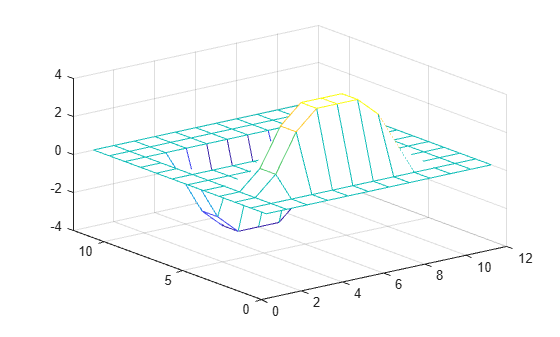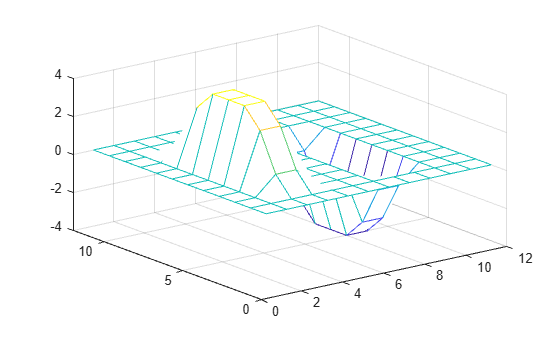# conv2

## 语法

``C = conv2(A,B)``
``C = conv2(u,v,A)``
``C = conv2(___,shape)``

## 说明

``C = conv2(A,B)` 返回矩阵 `A` 和 `B` 的二维卷积。`

``C = conv2(u,v,A)` 首先求 `A` 的各列与向量 `u` 的卷积，然后求每行结果与向量 `v` 的卷积。`

``C = conv2(___,shape)` 根据 `shape` 返回卷积的子区。例如，`C = conv2(A,B,'same')` 返回卷积中大小与 `A` 相同的中心部分。`

## 示例

```A = rand(3); B = rand(4); Cfull = conv2(A,B)```
```Cfull = 6×6 0.7861 1.2768 1.4581 1.0007 0.2876 0.0099 1.0024 1.8458 3.0844 2.5151 1.5196 0.2560 1.0561 1.9824 3.5790 3.9432 2.9708 0.7587 1.6790 2.0772 3.0052 3.7511 2.7593 1.5129 0.9902 1.1000 2.4492 1.6082 1.7976 1.2655 0.1215 0.1469 1.0409 0.5540 0.6941 0.6499 ```

`Csame = conv2(A,B,'same')`
```Csame = 3×3 3.5790 3.9432 2.9708 3.0052 3.7511 2.7593 2.4492 1.6082 1.7976 ```

Sobel 求边运算利用二维卷积来检测图像的边和其他二维数据。

```A = zeros(10); A(3:7,3:7) = ones(5); mesh(A)``````u = [1 0 -1]'; v = [1 2 1]; Ch = conv2(u,v,A); mesh(Ch)``````Cv = conv2(v,u,A); mesh(Cv)``````figure mesh(sqrt(Ch.^2 + Cv.^2))```## 输入参数

• `'full'` - 返回完整的二维卷积。

• `'same'` - 返回卷积中大小与 `A` 相同的中心部分。

• `'valid'` - 仅返回计算的没有补零边缘的卷积部分。

## 输出参数

`conv2` 的一个或多个输入参数的类型为 `single` 时，输出的类型为 `single`。否则，`conv2` 会将输入转换为类型 `double`，并返回类型 `double`

## 详细信息

### 二维卷积

`$C\left(j,k\right)=\sum _{p}\sum _{q}A\left(p,q\right)B\left(j-p+1,k-q+1\right)$`

p 和 q 会遍历所有可得到 A(p,q) 和 B(j-p+1,k-q+1) 的合法下标的值。